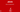# Else if statement in JavaIf statement is used to single condition. But if we want to validate multiple condition then we can use else if. If else is used if we have multiple condition and choose 1 condition at a time. For example, if we want to validate that, if a = 0 then print Zero, if a = 1 then print one, if a = 2 then print two and so on.

In if else statement only one block will be executed. Which means only 1 condition will be true at a time so code of that block will be executed at that time.

So the first IF tests for condition number one (18 or under, for example). Next comes else if, followed by a pair of round brackets. The second condition goes between these new round brackets. Anything not caught by the first two conditions will be caught be the final else. Again, code is sectioned off using curly brackets, with each if, else if, or else having its own pair of curly brackets. Miss one out and you’ll get error messages.

Before trying out some new code, you’ll need to learn some more conditional operators. The ones you have used so far are these:

> Greater Than
< Less Than
>= Greater Than or Equal To
<= Less Than or Equal To

Here’s four more you can use:

&& AND
|| OR
== HAS A VALUE OF
! NOT  

The first one is two ampersand symbols, and is used to test for more than one condition at the same time. We can use it to test for two age ranges:

else if (user > 18 && user < 40)  

Here, we want to check if the user is older than 18 but younger than 40. Remember, we’re trying to check what is inside of the user variable. The first condition is “Greater than 18” (user > 18). The second condition is “Less than 40” (user < 40). In between the two we have our AND operator (&&). So the whole line says “else if user is greater than 18 AND user is less than 40.”

The various conditional operators can be tricky to use. But you’re just testing a variable for a particular condition. It’s simply a question of picking the right conditional operator or operators for the job.